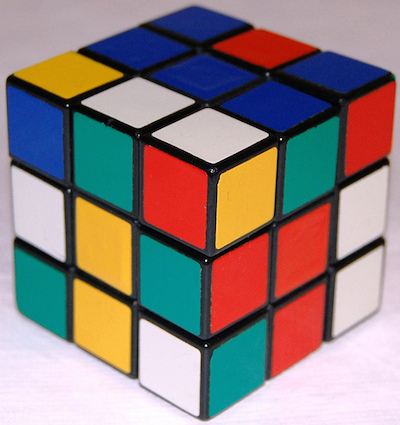# Rubik Madness

Probability Level 1If you take a $3 \times 3 \times 3$ Rubik cube and break it up into the $3^3$ cubes, how many cubes would have stickers on exactly $2$ faces?

Bonus question: Can you generalize this for a $N \times N \times N$ Rubik cube?

×

Problem Loading...

Note Loading...

Set Loading...